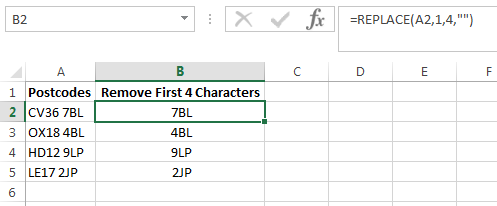Our Recent Posts

Archive

Tags

No tags yet.

# How to Remove First 4 Characters in Excel

You may have a workbook which contains text but you only want to extract certain characters from it. For example, you may only want to extract the first name from a cell which contains the first and last name of a person. In this tutorial I will show you how to manipulate text and in particular I will show you some great techniques on how to remove the first 4 characters in Excel using the following functions:

1) Excel RIGHT Function

2) Excel MID Function

3) Excel REPLACE Function

### Using the Excel RIGHT FunctionIn this example I have a list of UK postcodes. I want to remove the first 4 characters to leave just the last 3 characters of the postcode.The formula in cell B2 is =RIGHT(A2,LEN(A2)-4). I then copied the formula down using the fill handle.

### How does this Formula Work?

So how does this formula work? Let’s break this down so you can understand how it calculates. I will use the formula in cell B2 for the explanation.

RIGHT Function

The RIGHT function extracts a given number of characters from the right side of a specified text. For example =RIGHT(“bananas”,4) will result in “anas”

LEN Function

The LEN function extracts the length of a given string. For example =LEN(“apples”) will result in 6 as there are 6 characters in the string “apples”.

RIGHT(A2,LEN(A2)

This section of the formula will return 8. For the first argument of the RIGHT function you have to specify what text to use. In this example it is cell A2. For the second argument you have to specify the number of characters you want to extract. For this argument I am using the LEN function which returns the number of characters of the postcode CV36 7BL which is 8. The space between CV36 and 7BL counts as a character. The formula =RIGHT(A2,LEN(A2) translates to =RIGHT(A2,8) which returns CV36 7BL.

RIGHT(A2,LEN(A2)-4)

I want to remove the first 4 characters so therefore I include a -4 at the end of the formula. LEN(A2)-4 therefore returns 4 (8-4=4).

If I simplify this further the RIGHT function is =RIGHT(A2,4) and returns CV36.

### How do you Remove the First nth Character of a String?If you want to remove the first nth characters in a string you just change the -4 to whatever number of characters you want to remove. For example, if you want to remove the first 3 characters of a string then simply change the -4 to -3. If you want to remove the first 2 characters then change it to -2 and so on.

### Using the Excel MID FunctionThe formula in cell B2 is =MID(A2,5,LEN(A2)). I then just copied the formula down using the fill handle.

### How does this Formula Work?

I will explain how the formula in cell B2 works.

MID Function

The Excel MID function extracts the middle of a text based on the specified number of characters. For example, =MID("bananas",3,2) returns “na”. The first argument is the text string or a cell reference you want to extract from. The second argument is the first character you want to extract. The third argument is the number of characters you want to extract.

=MID(A2,5

This section of the formula is saying start from the fifth character of the postcode CV36 7BL. This means that it will start from the space as the space is the fifth character along from the left.

LEN(A2)

The LEN function is returning the number of characters of the postcode CV36 7BL which is 8.

=MID(A2,5,LEN(A2))

If you simplify this formula the MID function is =MID(A2,5,8). It starts from the space and extracts 8 characters along. Because there is only 3 characters after the space it therefore extracts 7BL.

### How do you Remove the First nth Character of a String?If you want to remove the first nth character then just add a 1 in the MID functions second argument. For example if I want to remove the first 3 characters then I enter 4 in the MID functions second argument. If I want to remove the first 2 characters then just enter 3 for the second argument.

### Using the Excel REPLACE FunctionThe formula in cell B2 is =REPLACE(A2,1,4,""). I then copied the formula down using the fill handle.

### How does this Formula Work?

I will explain how this formula works using the formula in cell B2 as an example.

The REPLACE Function

The REPLACE function replaces a set of characters in a string with another set of characters. The first argument of the replace function is the string or the cell you want to replace characters with. The second argument is the position of the old text to begin replacing characters. The third argument is the number of characters you want to replace the old text with. The fourth argument is the new characters you want to replace the old text with.

REPLACE(A2,1,4,"")

The first argument is the postcode in cell A2. The second argument is the start number. I want to start from the beginning so I enter a 1. The third argument is 4 as I want to replace the first 4 characters with new text. The last argument is two quotation marks which mean a blank string. I want to replace the first 4 characters with a blank string so I am left with the last 3 characters.

### How do you Remove the First nth Character of a String?To remove the first nth character just replace the third argument with the number of characters you want to remove. For example, if you want to remove the first 3 characters then just change the third argument to 3.

I hope you enjoyed this tutorial on how to remove the first 4 characters in Excel. If you have any questions or feedback then please leave a comment below.